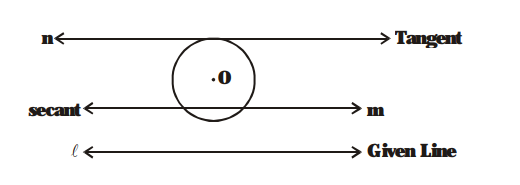# Draw a circle and two lines parallel to a given

Question:

Draw a circle and two lines parallel to a given line such that one is tangent and other a secant to the circle.

Solution:

We have the required figure, as shownHere, $\ell$ is the given line and a circle with centre $\mathrm{O}$ is drawn.

The line $\mathrm{n}$ is drawn which is parallel to $\ell$ and tangent to the circle. Also, $\mathrm{m}$ is drawn parallel to line $\ell$ and is a secant to the circle.